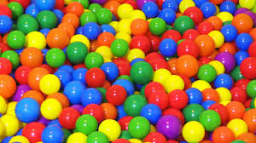# Probability bullets

There are six red, five green, eight blue, 11 yellow balls in the bag. What is the probability that we will pull out the green bullet?

p =  0.1667

### Step-by-step explanation:Did you find an error or inaccuracy? Feel free to write us. Thank you!

Showing 1 comment:
Math student
Should read "What is the probability that we will pull out a green ball?"

1 month ago  1 Like

Tips to related online calculators
Need help to calculate sum, simplify or multiply fractions? Try our fraction calculator.
Would you like to compute count of combinations?